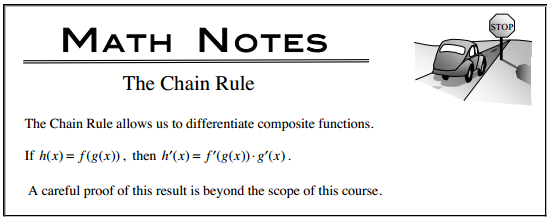### Home > CALC > Chapter 5 > Lesson 5.2.2 > Problem5-74

5-74.

Chris found the derivative of $(2x + 5)^2$ as shown below.

$\frac { d } { d x } ( ( 2 x + 5 ) ^ { 2 } ) = \frac { d } { d x } ( 4 x ^ { 2 } + 20 x + 25 ) = 8 x + 20$

To check his solution, he tried applying the Power Rule:

$\frac { d } { d x } ( ( 2 x + 5 ) ^ { 2 } ) = 2 ( 2 x + 5 ) = 4 x + 10$

His solutions did not match! Which is the correct derivative, and why?